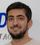cancel
Showing results for
Did you mean:Frequent Visitor

## DAX formula to return a Boolean value for previous quarter in calculated column

Hi, I've been puzzling over this for some time.  I'd like to use a column called "Previous Quarter" in my Calendar.  I was able to back into current quarter, but I'm having a heck of a time with this one.  Can anyone help?1 ACCEPTED SOLUTIONSolution Sage

Hi @rdwhite ,

Follow Steps Below (Creating Calculated Columns) assuming my date table name is FullDDate:

1-Create Calculated Column:

YearQuarterNo =
CONVERT (
CONCATENATE (
YEAR ( FullDDate[Date] ),
CONCATENATE ( "0", FullDDate[Quarter] )
),
INTEGER
)

2-Then create calculated column below:

YearQuarterIndex =
RANKX (
FullDDate,
FullDDate[YearQuarterNo],
FullDDate[YearQuarterNo],
ASC,
DENSE
)

AS your FInal step, create the calculated column below to get what you need:

PreviousQuarter =
IF (
FullDDate[YearQuarterIndex]
CALCULATE (
MIN ( FullDDate[YearQuarterIndex] ) - 1,
FILTER ( FullDDate, FullDDate[Date] = TODAY () )
),
"YES",
"NO"
)

If this answer solves your problem, please give it a thumbs up and mark it as an accepted solution so the others would find what they need easier.

Regards,
Loran

9 REPLIES 9Frequent Visitor

Here is what I've tried without sucess so far: "Previous Quarter Boolean = VAR PrevQuarter = DATEADD('Calendar'[Date],-1,QUARTER) RETURN IF(YEAR(PrevQuarter) = YEAR('Calendar'[Date]) && QUARTER(PrevQuarter) = QUARTER('Calendar'[Date]), TRUE(), FALSE() ) "  All returned False.  Also: "Previous Quarter Boolean = VAR PrevQuarterStartDate = DATE(YEAR('Calendar'[Date]), SWITCH(QUARTER('Calendar'[Date]), 1, 10, 2, 1, 3, 4, 4, 7), 1) VAR PrevQuarterEndDate = EOMONTH(PrevQuarterStartDate, 2) RETURN IF('Calendar'[Date] >= PrevQuarterStartDate && 'Calendar'[Date] <= PrevQuarterEndDate, TRUE(), FALSE() )"Solution Sage

Have you checked my answer? I think it is what you need.Frequent Visitor

The first step did not work...Solution Sage

This is a calculated column as well:

quarter=QUARTER(fullddate[date])

Then it is used as one of the inputs in step1.Solution Sage

You are doing sth wrong.

Are you creating a calculated column? What is the error?Frequent VisitorSolution Sage

fullddate[quarter] in my formula is just number. 1,2,3,4 (without qtr string)

You can make it using a calculated column:

quarter=QUARTER(fullddate[date])Solution Sage

Hi @rdwhite ,

Follow Steps Below (Creating Calculated Columns) assuming my date table name is FullDDate:

1-Create Calculated Column:

YearQuarterNo =
CONVERT (
CONCATENATE (
YEAR ( FullDDate[Date] ),
CONCATENATE ( "0", FullDDate[Quarter] )
),
INTEGER
)

2-Then create calculated column below:

YearQuarterIndex =
RANKX (
FullDDate,
FullDDate[YearQuarterNo],
FullDDate[YearQuarterNo],
ASC,
DENSE
)

AS your FInal step, create the calculated column below to get what you need:

PreviousQuarter =
IF (
FullDDate[YearQuarterIndex]
CALCULATE (
MIN ( FullDDate[YearQuarterIndex] ) - 1,
FILTER ( FullDDate, FullDDate[Date] = TODAY () )
),
"YES",
"NO"
)

If this answer solves your problem, please give it a thumbs up and mark it as an accepted solution so the others would find what they need easier.

Regards,
LoranFrequent Visitor

Thanks for stepping through this with me.  I had even resulted to ChatGPT...